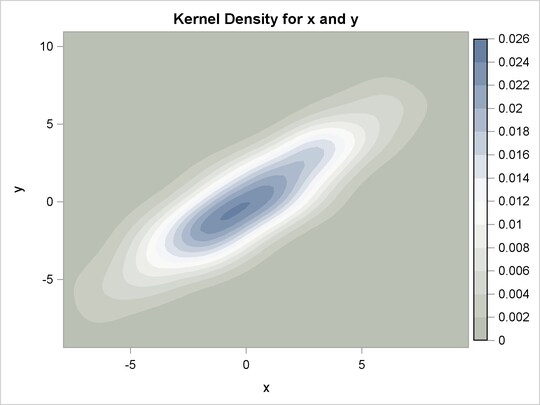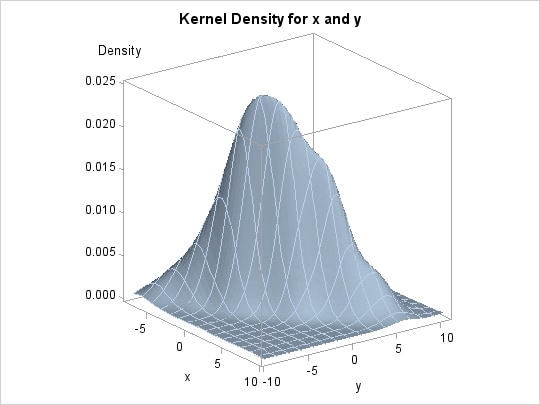Statistical Graphics Using ODS
 Contour and Surface Plots with PROC KDE

This example is taken from the section Getting Started: KDE Procedure in Chapter 45, The KDE Procedure. Here, in addition to the ODS GRAPHICS statement, procedure options are used to request plots. The following statements simulate 1,000 observations from a bivariate normal density with means (0,0), variances (10,10), and covariance 9:

```data bivnormal;
do i = 1 to 1000;
z1 = rannor(104);
z2 = rannor(104);
z3 = rannor(104);
x  = 3*z1+z2;
y  = 3*z1+z3;
output;
end;
run;
```

The following statements request a bivariate kernel density estimate for the variables x and y:

```ods graphics on;

proc kde data=bivnormal;
bivar x y / plots=contour surface;
run;
```

The PLOTS= option requests a contour plot and a surface plot of the estimate (displayed in Figure 21.5 and Figure 21.6, respectively). The results are displayed in the STATISTICAL style. For more information about the graphics available in PROC KDE, see the section ODS Graphics of Chapter 45, The KDE Procedure.

Figure 21.5 Contour Plot of Estimated DensityFigure 21.6 Surface Plot of Estimated DensityPrevious Page | Next Page | Top of Page